Next: F.4 Temporal Gain Correction Up: Calibration Files for Standard Previous: F.2 Correction for ADC

# F.3 Gain Saturation Correction (GSC)

At large charge input (high energy photons as well as high count rate) some saturation of the gain is arising in the PSPC. The fall-off in the signal amplification as a function of event amplitude has been modeled using spectral calibration data (cf. Eq. (F.6)) . At present the rate gain effect is not taken into account.

The offset values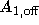for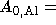135, 146, and 103 are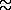89.2, 96.1, and 69.1, respectively. Below these values thresholds gain saturation is negligible.

According to Chap. 3 the rate gain effect can be understood in terms of pseudo-continuous presence of a substantial electron cloud causing a reduction in the electronic field. Measurements at the PANTER test facility have shown a drop of the gain of 1% at E = 1.487keV and 2% at E = 3keV for an X-ray count rate of 500s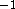. Further investigations were planned.

Figure F.3 shows the PHA channel difference between the non-linearity and gain saturation correction.Figure F.3: Difference between the channels after gain saturation correction (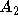) and after non-linearity correction (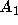) as function ofaccording to Eq. (F.6). The correction effect is shown for three typical gain values (, 135, 146).Parameters: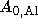] PHA channel of the Al K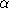line (BAL ) (from Prescott fit)pulse height of event, corrected for ADC non-linearitypulse height of event, corrected for ADC non-linearity and gain saturation

SASS source code: package PSPC, bubble CT, subroutine CGS

SASS files: none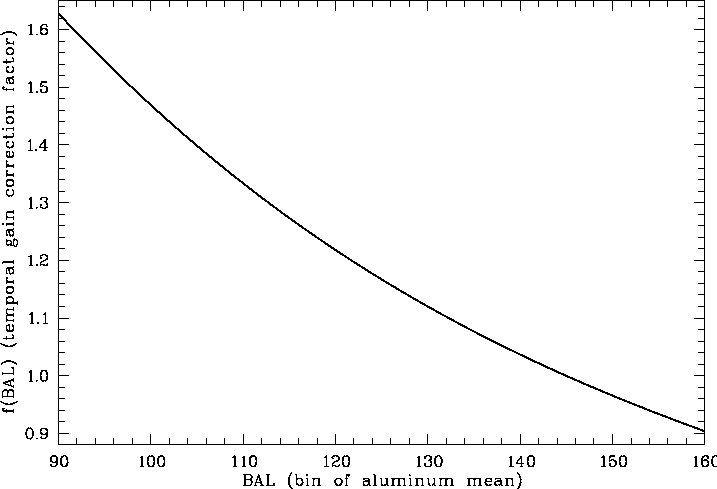Figure F.4: The temporal gain correction factor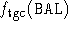according to Eq.(F.7) as function of the gain (expressed in terms of the Al Kline bin). It is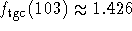,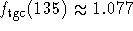, and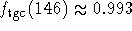.

If you have problems/suggestions please send mail to rosat_svc@mpe-garching.mpg.de